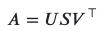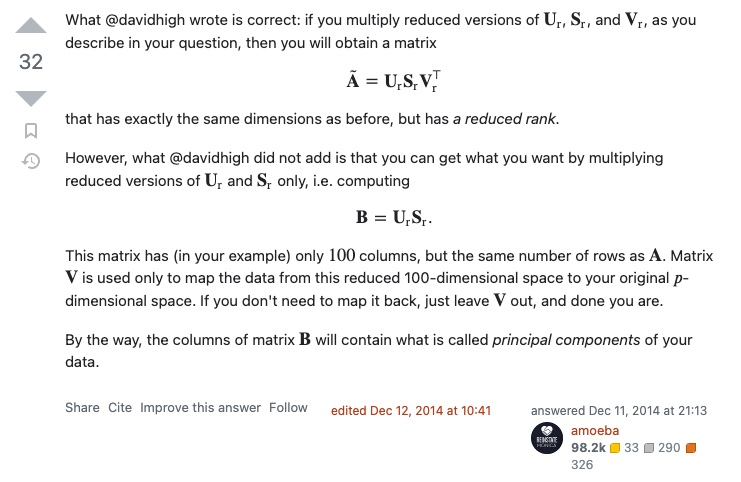# How to use SVD for dimensionality reduction to reduce the number of columns (features) of the data matrix?

From: https://stats.stackexchange.com/questions/107533/how-to-use-svd-for-dimensionality-reduction-to-reduce-the-number-of-columns-fea

My original data has many more columns (features) than rows (users). I am trying to reduce the features of my SVD (I need all of the rows). I found one method of doing so in a book called “Machine Learning in Action” but I don’t think it will work for the data I am using.

The method is as follows. Define SVD asSet an optimization threshold (i.e., 90%). Calculate the total sum of the squares of the diagonal ? matrix. Calculate how many ? values it takes to reach 90% of the total sum of squares. So if that turns out to be 100 ? values, then I would take the first 100 columns of the ? matrix, first 100 rows of the ?^ matrix, and a 100×100100×100 square matrix out of the ? matrix. I would then calculate ?=???^ using the reduced matrices.

However, this method does not target the columns of my original data, since the dimensions of the resulting ? matrix are the same as before. How would I target the columns of my original matrix?